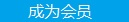您可以捐助，支持我们的公益事业。 1元 10元 50元 认证码：必填求知 文章 文库 Lib 视频 Code iProcess 课程 认证 咨询 工具 火云堂 讲座吧 成长之路要资料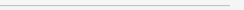订阅捐助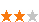116 次浏览     评价： 好 中 差
2019-3-7

1）给数据中的每一个样本一个权重

2）训练数据中的每一个样本，得到第一个分类器

3）计算该分类器的错误率，根据错误率计算要给分类器分配的权重（注意这里是分类器的权重）

4）将第一个分类器分错误的样本权重增加，分对的样本权重减小（注意这里是样本的权重）

5）然后再用新的样本权重训练数据，得到新的分类器，到步骤3

6）直到步骤3中分类器错误率为0，或者到达迭代次数

7）将所有弱分类器加权求和，得到分类结果（注意是分类器权重）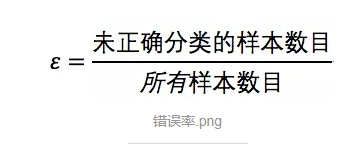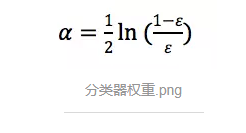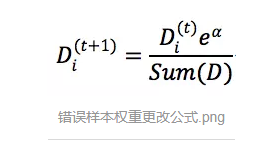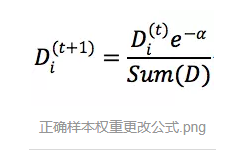import numpy as np def loadSimpData(): datMat = np.matrix([[1. , 2.1], [1.5 , 1.6], [1.3, 1. ], [1. , 1. ], [2. , 1. ]]) classLabels = [1.0, 1.0, -1.0, -1.0, 1.0] return datMat, classLabels datMat, classLabels = loadSimpData() showScatter(datMat, classLabels)

showScatter方法用来绘制散点图，代码如下：

 import matplotlib.pyplot as plt def showScatter(matrix, labels): plt.figure(figsize=(8,6)) x1 = []; y1 = []; x2 = []; y2 = [] for i in range(len(labels)): if labels[i] == 1.0: x1.append(matrix[i, 0]) y1.append(matrix[i, 1]) else: x2.append(matrix[i, 0]) y2.append(matrix[i, 1]) plt.scatter(x1, y1, marker='o', color='green', alpha=0.7, label='1.0') plt.scatter(x2, y2, marker='^', color='red', alpha=0.7, label='-1.0') plt.title('dataset') plt.ylabel('variable Y') plt.xlabel('Variable X') plt.legend(loc='upper right') plt.show()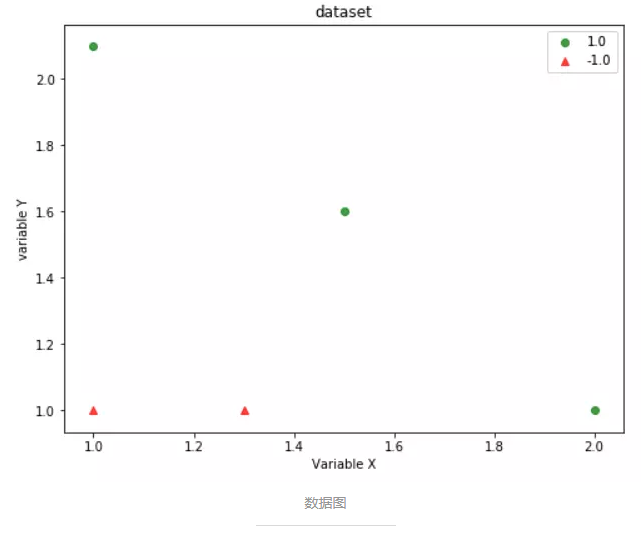# [[5, 5], [0, 0]]是要分类的点 # classifierArr是弱分器数组 adaClassify ([[5, 5], [0, 0]], classifierArr)

[[1.], [-1.]]

 # datToClass：要分类的数据 # classifierArr：弱分类器数组 def adaClassify(datToClass, classifierArr): dataMatrix = np.mat(datToClass) m = np.shape(dataMatrix) aggClassEst = np.mat(np.zeros((m, 1))) # 为了满足输出期望，先用0列向量初始化输出结果 for i in range(len(classifierArr)): classEst = stumpClassify(dataMatrix, classifierArr[i]['dim'], classifierArr[i]['thresh'], classifierArr[i]['ineq']) # 得到一个弱分类器分类结果 aggClassEst += classifierArr[i]['alpha'] * classEst # 对应算法流程的步骤7，将弱分类器结果加权求和 # print(aggClassEst) return np.sign(aggClassEst) #由于是二类问题，所以可以根据加权求和结果的正负情况得到期望的分类输出

 # dataMatirx：要分类的数据 # dimen：维度 # threshVal：阈值 # threshIneq：有两种，‘lt’=lower than，‘gt’=greater than def stumpClassify(dataMatrix, dimen, threshVal, threshIneq): retArray = np.ones((np.shape(dataMatrix), 1)) if threshIneq == 'gt': retArray[dataMatrix[:, dimen] <= threshVal] = -1.0 # 如果希望大于阈值的是1，则小于阈值的部分置为-1 else: retArray[dataMatrix[:, dimen] > threshVal] = -1.0 return retArray

 def adaBoostTrainDS(dataArr, classLabels, numIt=40): weakClassArr = [] m = np.shape(dataArr) D = np.mat(np.ones((m,1)) / m) # 初始化权重向量，给每个样本相同的权重，[[1/m],[1/m],[1/m],...] aggClassEst = np.mat(np.zeros((m,1))) # 初始化每个样本的预估值为0 for i in range(numIt): # 遍历迭代次数 bestStump, error, classEst = buildStump(dataArr, classLabels, D) # 构建一棵单层决策树，返回最好的树，错误率和分类结果 alpha = float(0.5 * np.log((1.0 - error)/error)) #计算分类器权重 bestStump['alpha'] = alpha #将alpha值也加入最佳树字典 weakClassArr.append(bestStump) # 将弱分类器加入数组 # print("classEst:", classEst.T) # 更新权重向量D expon = np.multiply(-1*alpha*np.mat(classLabels).T, classEst) D = np.multiply(D, np.exp(expon)) D = D / D.sum() # 累加错误率，直到错误率为0或者到达迭代次数 aggClassEst += alpha * classEst print("aggClassEst:", aggClassEst.T) aggErrors = np.multiply(np.sign(aggClassEst) != np.mat(classLabels).T, np.ones((m, 1))) errorRate = aggErrors.sum() / m print("total error:", errorRate, "\n") if errorRate == 0.0: break; return weakClassArr

 # dataArr: 数据集 # classLabels：标签 # D：由每个样本的权重构成的矩阵 def buildStump(dataArr, classLabels, D): dataMatrix = np.mat(dataArr) labelMat = np.mat(classLabels).T # 标签转成列向量 m, n = np.shape(dataMatrix) #m为数据个数，n为每条数据含有的样本数（也就是特征） numSteps = 10.0 bestStump = {} bestClasEst = np.mat(np.zeros((m, 1))) # 初始化最好的分类器为[,,,...] minError = np.inf #最小错误率，不停更新最小错误率 for i in range(n): #遍历特征 rangeMin = dataMatrix[:, i].min(); # 找这一列特征的最小值 rangeMax = dataMatrix[:, i].max(); # 找这一列特征的最大值 stepSize = (rangeMax - rangeMin) / numSteps #每次移动的步长 for j in range(-1, int(numSteps) + 1): #对每个步长 for inequal in ['lt', 'gt']: # 每个条件，大于阈值是1还是小于阈值是1 threshVal = (rangeMin + float(j) * stepSize) # 阈值设为最小值+第j个步长 print('i=%d, threshVal=%f, inequal=%s'%(i,threshVal,inequal)) predictedVals = stumpClassify(dataMatrix, i , threshVal, inequal) # 将dataMatrix的第i个特征inequal阈值的置为1，否则为-1 print(predictedVals) errArr = np.mat(np.ones((m, 1))) errArr[predictedVals == labelMat] = 0 # 预测对的置0 print(errArr) weightedError = D.T * errArr print("split: dim %d, threshold %.2f, threshold inequal: %s, the weighted error is %.3f" % (i, threshVal, inequal, weightedError)) if weightedError < minError: minError = weightedError bestClasEst = predictedVals.copy() bestStump['dim'] = i bestStump['thresh'] = threshVal bestStump['ineq'] = inequal return bestStump, minError, bestClasEst

 # 分类（0，0）点 adaClassify([0,0], classifierArray)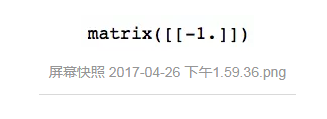116 次浏览  评价: 好 中 差订阅捐助
 相关文章 我们该如何设计数据库 数据库设计经验谈 数据库设计过程 数据库编程总结
 相关文档 数据库性能调优技巧 数据库性能调整 数据库性能优化讲座 数据库系统性能调优系列
 相关课程 高性能数据库设计与优化 高级数据库架构师 数据仓库和数据挖掘技术 Hadoop原理、部署与性能调优每天2个文档/视频 扫描微信二维码订阅
 订阅技术月刊 获得每月300个技术资源